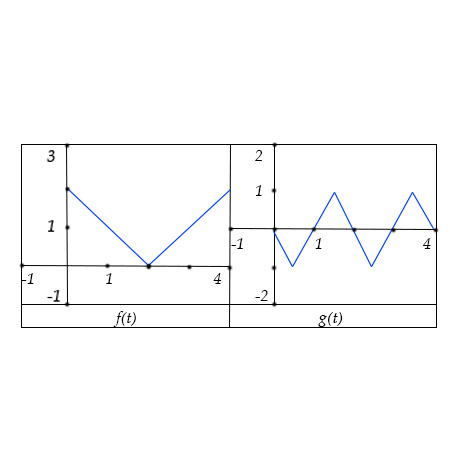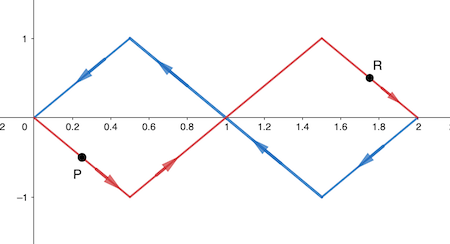# The functions f(t) and g(t) are shown below. If the motion of a particle whose position at time t...

## Question:

The functions {eq}f(t) {/eq} and {eq}g(t) {/eq} are shown below.

If the motion of a particle whose position at time {eq}t {/eq} is given by {eq}x=f(t), y=g(t), {/eq} sketch a graph of the resulting motion and use your graph to answer the following questions:

a. What is the slope of the graph at {eq}(1.75, 0.5) {/eq}?

b. At this point, where is the particle moving?

c. What is the slope of the graph at {eq}(0.25,-0.5) {/eq}?

d. At this point, where is the particle moving?## Parametric Curves

A curve given parametrically as {eq}\displaystyle x=f(t), y=g(t), a\leq t\leq b, {/eq} is a curve whose points {eq}\displaystyle (x,y), {/eq} are obtained by giving values to {eq}\displaystyle t, {/eq} in an increasing order of {eq}\displaystyle t. {/eq}

The direction of a parametric curve is given by the direction of increasing {eq}\displaystyle t. {/eq}

Sometimes, to plot a parametric curve, we convert the curve in Cartesian form, by eliminating the parameter {eq}\displaystyle t, {/eq} and obtaining a relationship between {eq}\displaystyle x\text{ and } y. {/eq}

If we have the graphs of the two functions, {eq}\displaystyle f(t), g(t) {/eq} then we analyze the direction of the curve in Cartesian coordinates, by tracing the direction of the two coordinates for different values of {eq}\displaystyle t. {/eq}

To find the slope of the curve in Cartesian coordinates, {eq}\displaystyle \frac{dy}{dx} {/eq} we can obtain the slopes of the two functions {eq}\displaystyle f'(t), g'(t) {/eq} and use {eq}\displaystyle \frac{dy}{dx} =\frac{g'(t)}{f'(t)}. {/eq}

To sketch the graph of the parametric curve {eq}\displaystyle x=f(t), y=g(t), 0\leq t\leq 4 {/eq} shown in the graphs, we will plot the points {eq}\displaystyle (x,y) {/eq} for values of {eq}\displaystyle t {/eq} in increasing order, to find also the direction of the curve.

For example, for {eq}\displaystyle t=0: x=2,y=0, {/eq} the {eq}\displaystyle x- {/eq} coordinate decreases linearly until {eq}\displaystyle t=2, {/eq} while the {eq}\displaystyle y- {/eq} coordinate decreases linearly up to {eq}\displaystyle t=0.5, \text{corresponding to } x=1.5 \text{ and } y=-1. {/eq}

Continuing this analysis we obtain the graph shown below by the blue and red solid lines.a. The slope of the graph at the point {eq}\displaystyle R(1.75, 0.5) {/eq} which corresponds to the interval where {eq}\displaystyle x(t) {/eq} is increasing and {eq}\displaystyle y(t) {/eq} is decreasing, so for {eq}\displaystyle t\in [3.5,4] {/eq} where {eq}\displaystyle x'(t)=1 \text{ and } y'(t)= -2, {/eq}

the slope is given by the ratio of {eq}\displaystyle \frac{dy}{dx}=\frac{y'(t)}{x'(t)}=\boxed{-2 }. {/eq}

b. The value {eq}\displaystyle t {/eq} corresponding to the point {eq}\displaystyle R(1.75, 0.5) {/eq} is the common value {eq}\displaystyle t {/eq}

of the intersection of the graph of {eq}\displaystyle x=f(t) {/eq} with the horizontal line {eq}\displaystyle x=1.75, {/eq} and the intersection of the graph of {eq}\displaystyle y=g(t) {/eq} with the horizontal line {eq}\displaystyle y=0.5 {/eq}

so, the value of {eq}\displaystyle t {/eq} is in the interval {eq}\displaystyle [3.5,4]. {/eq}

Because on {eq}\displaystyle [3.5,4], {/eq} {eq}\displaystyle y- {/eq} coordinate is decreasing and {eq}\displaystyle x- {/eq} coordinate, is increasing

{eq}\displaystyle \boxed{\text{ the point is moving toward }t=4: x=2 \text{ and } y=0}. {/eq}

c. The value {eq}\displaystyle t {/eq} corresponding to the point {eq}\displaystyle P(0.25, -0.5) {/eq} is the common value {eq}\displaystyle t {/eq}

of the intersection of the graph of {eq}\displaystyle x=f(t) {/eq} with the horizontal line {eq}\displaystyle x=0.25, {/eq} and the intersection of the graph of {eq}\displaystyle y=g(t) {/eq} with the horizontal line {eq}\displaystyle y=-0.5 {/eq}

so, the value of {eq}\displaystyle t {/eq} is in the interval {eq}\displaystyle [2,2.5]. {/eq}

Because on {eq}\displaystyle [2,2.5], {/eq} the {eq}\displaystyle x- {/eq} coordinate is increasing and the {eq}\displaystyle y- {/eq} coordinate is decreasing, with {eq}\displaystyle x'(t)=1 \text{ and } y'(t)= -2, {/eq}

then the slope is given by the ratio of {eq}\displaystyle \frac{dy}{dx}=\frac{y'(t)}{x'(t)}=\boxed{-2 }. {/eq}

d. Because on {eq}\displaystyle [2,2.5], {/eq} {eq}\displaystyle y- {/eq} coordinate is decreasing and {eq}\displaystyle x- {/eq} coordinate is increasing

{eq}\displaystyle \boxed{\text{ the point is moving toward }t=2.5: x=0.5 \text{ and } y=-1}. {/eq}